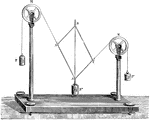### Gravesande's Apparatus

An apparatus for verifying the parallelogram of forces. This method is used in physics for determining…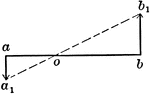### Motions Of 2 Points In Same Plane And Parallel

Illustration showing two points a and b to be in the same plane and parallel.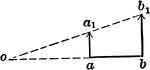### Motions Of 2 Points In Same Plane And Parallel

Illustration showing two points a and b to be in the same plane and parallel.### Motions Of 3 Points

Illustration showing three points a, b, and c in motion. The magnitude and direction of a and b are…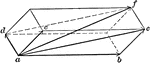### Parallelopiped of Motions

Illustration used "If three component motions ab, ac, and ad are combined, their resultant af will be…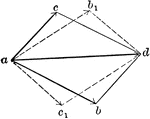### Vector Addition Given Resultant

Illustration "where ad is the given resultant, if the two components have the magnitudes represented…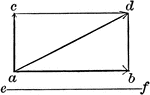### Vector Motion Into Two Components - Resultant

Illustration used to resolve a motion into two components, one of which is perpendicular, and the other…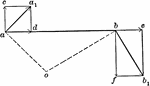### Velocities Of Rigidly-connected Points

Illustration for rigidly-connected points. "If two points are so connected that their distance apart…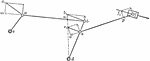### Velocities Of Rigidly-connected Points

Illustration for rigidly-connected points. In the series of links shown, c and d are fixed axes and…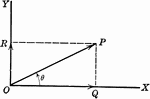### Vector

OP is a line representing a vector (directed quantity)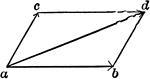Illustration showing two component forces ab and ac acting upon point a. The result is the vector ad…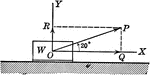### Vector Showing Force at Angle of 20 degrees

OP is a vector (directed quantity) showing the force by a weight acting on it at an angle of 20 degrees.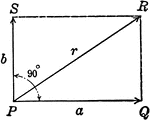### Resultant Vector With Vectors at 90 degrees

Illustration of the resultant vector when two vectors are acting upon a body at point P at 90 degrees.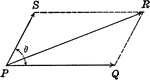### Resultant Vectors

Illustration of the resultant vector when two vectors are acting upon a body at point P.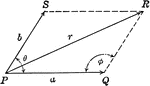### Resultant Vector With Vectors at Angle Ǝ

Illustration of the resultant vector when two vectors are acting upon a body at point P at Ǝ degrees.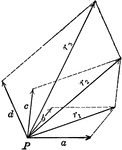### Resultant Vector From 4 Forces

Illustration of the resultant vector when four vectors are acting upon a body at point P.